Motors, batteries and meters

Voltage, current and resistance can be measured using meters.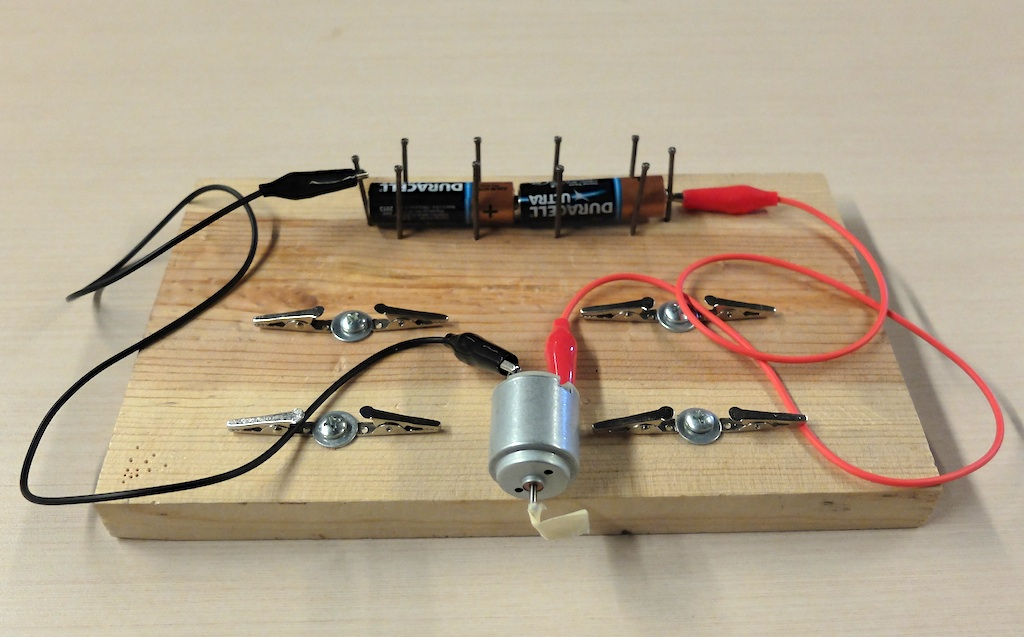A pine board with a battery holder made of nails and alligator clips is used to power a motor.

Material

• A small DC electric motor that will run on 3 volts. (Available for under \$2 from Pitsco or Kelvin)
• 2 x 1.5 volt AA batteries
• Two meters, one to measure voltage of 3 volts one to measure current up to 3 amps.
• A breadboard to connect the bulbs and batteries
• or a battery holder and alligator clips.

Assembly
Add a piece of tape to the shaft of a motor so you can see it rotate.
Attach the batteries
to the motor using alligator clips.

To Do and Notice

Notice that the voltmeter and ammeter should both have a red lead attached to their positive terminals and a black lead attached to their COM or common terminals.

Symbols

We'll use the symbol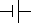for a battery or batteries, where the large plate indicates the positive end,

and the symbol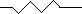for a motor or resistor.

Connect the motor to the batteries.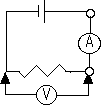Attach the voltmeter across the bulb.

Break the circuit and insert the ammeter between the battery and the bulb. (You can also do this one meter at a time.)

Notice that the voltmeter reads the voltage, probably between 2 and 3 volts.

Here is a meter measuring the voltage across the motor.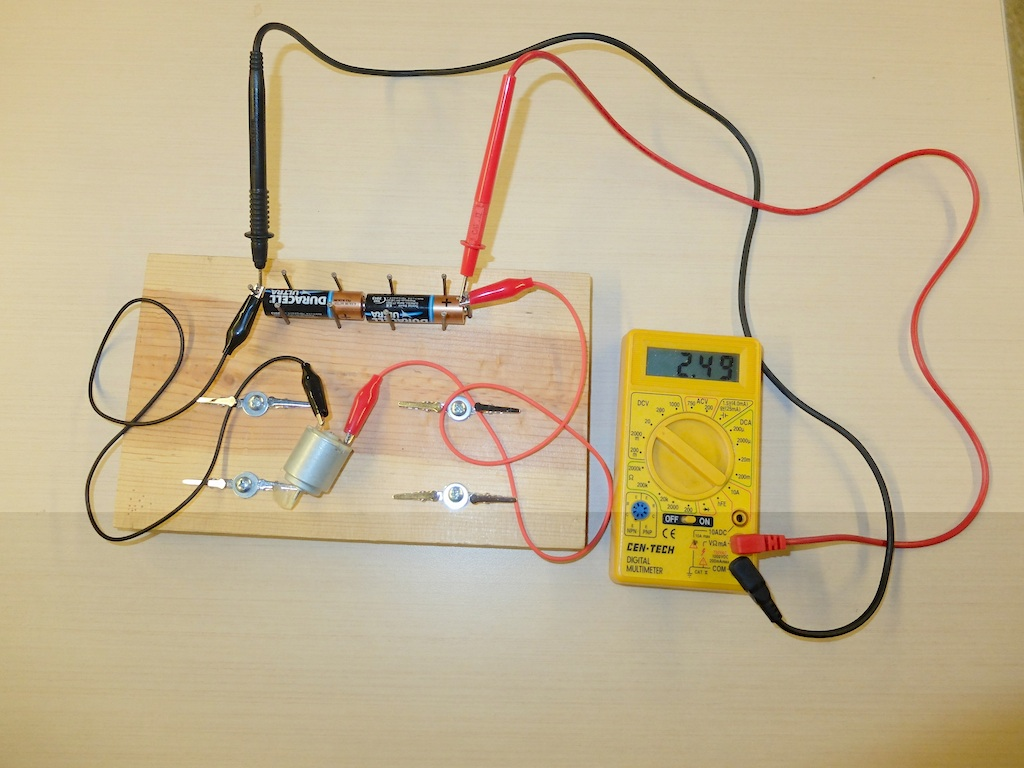A digital meter measures the voltage across the motor.

Here is a meter measuring the current through the motor.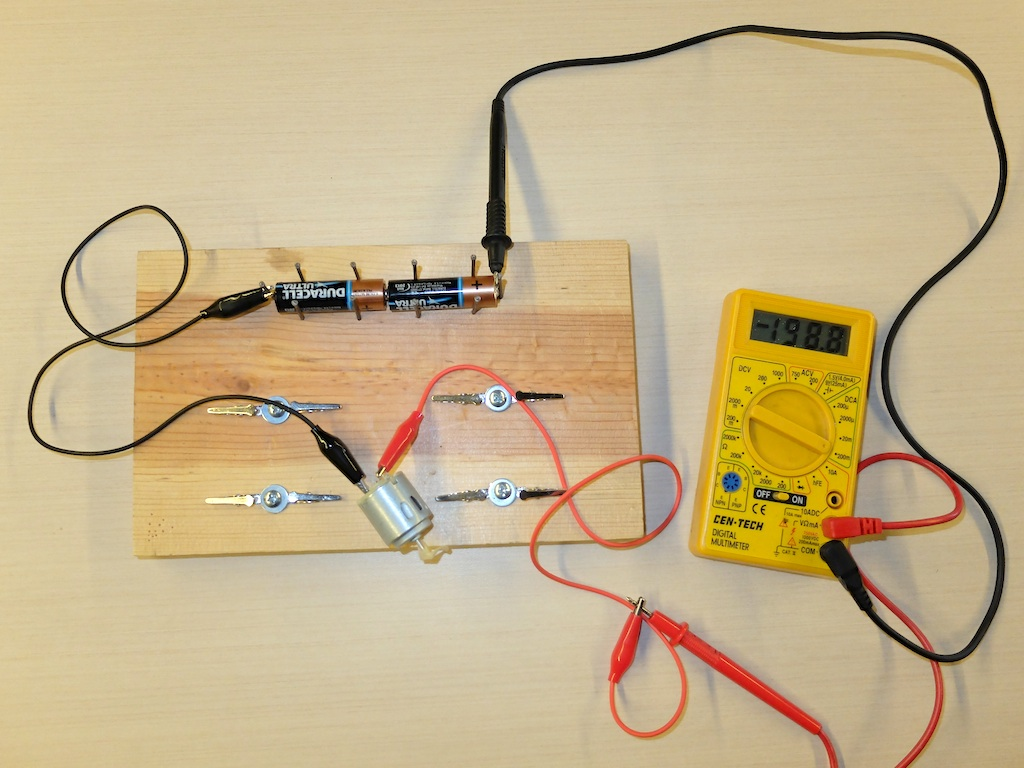Here is a meter inserted into the circuit to measure the current through the motor.

Grab the shaft of the motor and measure the voltage and current while the shaft is prevented from rotating.
Notice that the current increases greatly when the motor is stopped from rotating.(You will probably have to switch to the 10 Amp scale)

Math Root

You can calculate the resistance of the meter using R = V/I = 2.5 V/ 0.2A = 12 Ohms.

You can also calculate the power P = V * I = 2.5 V * 0.2 A = 0.5 watts.

When the motor stops spinning the current increases, for our motors it increased to 2 amps.

The new resistance is R = V/I = 2.5 V /2 A = 1.2 ohms

The new power is P = V *I = 2.5 * 2 = 5 watts

When the motor is stalled all the power goes into heat. Which can burn up a motor when it stalls.

What's Going On?

You When a current carrying wire in the motor moves near a magnet the magnet exerts a force on the wire which makes the motor spin.

However when a conducting wire moves near a magnet, whether there is a current going through it or not, the electrons in the wire experience a force. In the motor this electromotive force or EMF opposes the motion of the electrons created by the battery, so it is called a back EMF. This back EMF reduces the current and so increases the resistance of the motor when it is running.

When the motor stops there is no back EMF and so the current flows unopposed. The greater current causes high power dissipation in the motor.
 Scientific Explorations with Paul Doherty © 2015 7 August 2015